# How to Calculate and Solve for Shear Modulus of Rubber | Fracture Mechanics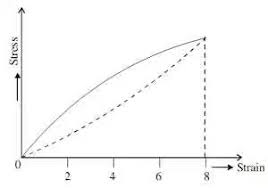The image above represents shear modulus of rubber.

To compute for shear modulus of rubber, three essential parameters are needed and these parameters are Density of Network Cross Links (N), Boltzmann’s Constant (KBand Temperature (T).

The formula for calculating shear modulus of rubber:

G = NKBT

Where:

G = Shear Modulus of Rubber
N = Density of Network Cross Links
KB = Boltzmann’s Constant
T = Temperature

Let’s solve an example;
Find the shear modulus of rubber when the density of network cross links is 21, the boltzmann’s constant is 1.38064852E-23 and the temperature is 10.

This implies that;

N = Density of Network Cross Links = 21
KB = Boltzmann’s Constant = 1.38064852E-23
T = Temperature = 10

G = NKBT
G = (21)(1.38064852e-23)(10)
G = 2.89

Therefore, the shear modulus of rubber is 2.89e-23 Pa.

Calculating the Density of Network Cross Links when the Shear Modulus of Rubber, Boltzmann’s Constant and the Temperature is Given.

N = G / KBT

Where;

N = Density of Network Cross Links
G = Shear Modulus of Rubber
KB = Boltzmann’s Constant
T = Temperature

Let’s solve an example;
Find the density of network cross links when the shear modulus of rubber is 14, the boltzmann’s constant is 1.380e-23 and temperature is 4.

This implies that;

G = Shear Modulus of Rubber = 14
KB = Boltzmann’s Constant = 1.380e-23
T = Temperature = 4

N = G / KBT
N = 14 / 1.380e-23 x 4
N = 14 / 5.52e-23
N = 2.54e-23

Therefore, the network cross links is 2.54e-23.

Calculating the Temperature when the Shear Modulus of Rubber, the Density of Network Cross Links and the Boltzmann’s Constant is Given.

T = G / KBN

Where;

T = Temperature
G = Shear Modulus of Rubber
N = Density of Network Cross Links
KB = Boltzmann’s Constant

Let’s solve an example;
Find the temperature when the shear modulus of rubber is 20, the density of network cross links is 18 and the boltzmann’s constant is 1.380e-23.

This implies that;

G = Shear Modulus of Rubber = 20
N = Density of Network Cross Links = 18
KB = Boltzmann’s Constant = 1.380e-23

T = G / KBN
T = 20 / 1.380e-23 x 18
T = 20 / 2.48e-22
T = 8.06e-22

Therefore, the temperature is 8.06e-22.

Nickzom Calculator – The Calculator Encyclopedia is capable of calculating the shear modulus of rubber.

To get the answer and workings of the shear modulus of rubber using the Nickzom Calculator – The Calculator Encyclopedia. First, you need to obtain the app.

You can get this app via any of these means:

To get access to the professional version via web, you need to register and subscribe for NGN 2,000 per annum to have utter access to all functionalities.
You can also try the demo version via https://www.nickzom.org/calculator

Apple (Paid) – https://itunes.apple.com/us/app/nickzom-calculator/id1331162702?mt=8
Once, you have obtained the calculator encyclopedia app, proceed to the Calculator Map, then click on Materials and Metallurgical under Engineering.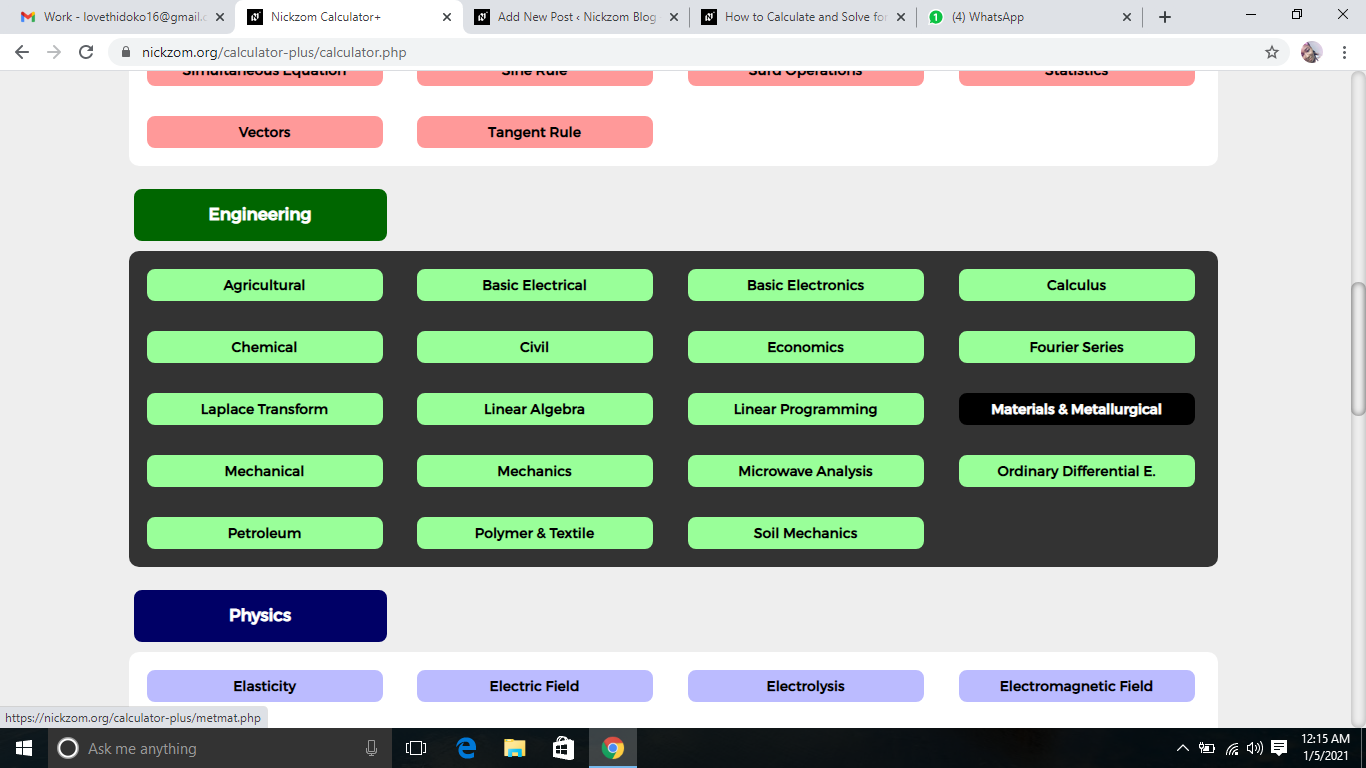Now, Click on Fracture Mechanics under Materials and MetallurgicalNow, Click on Shear Modulus of Rubber under Fracture Mechanics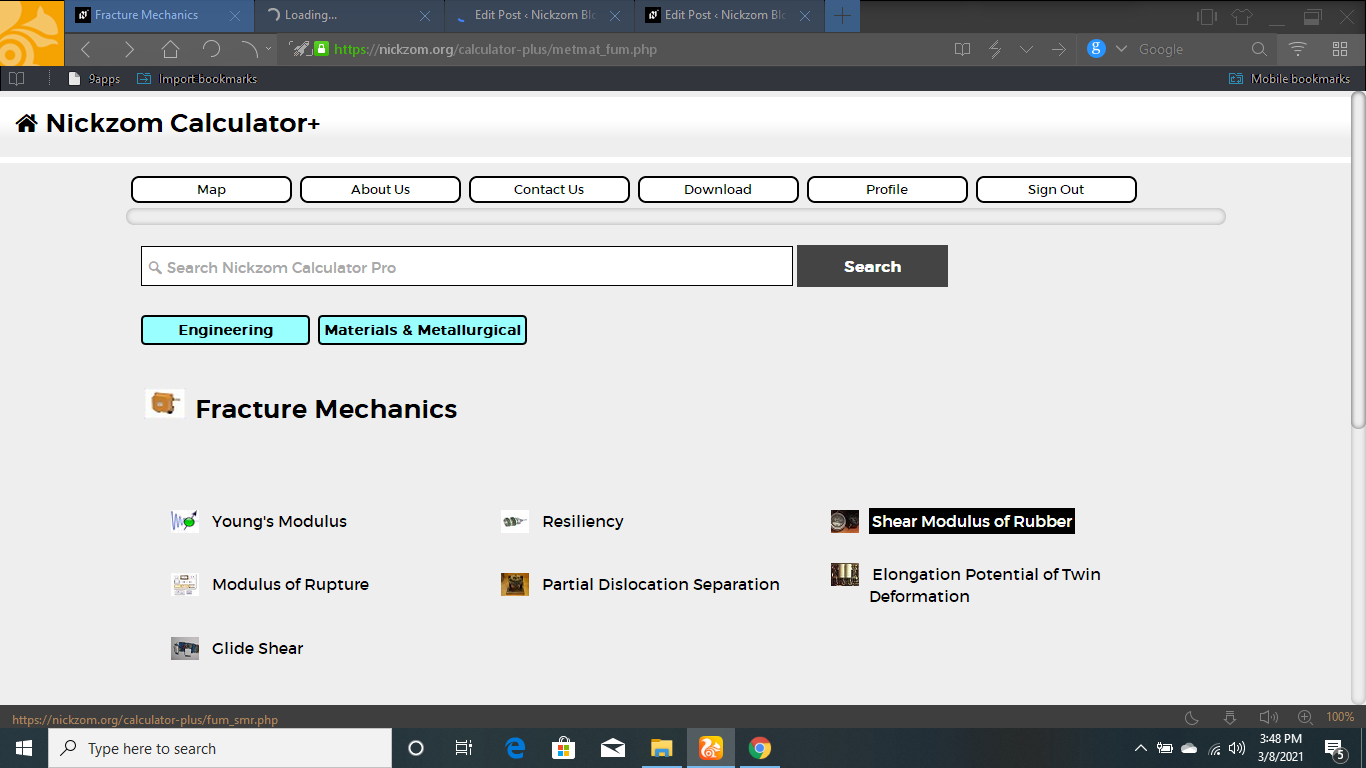The screenshot below displays the page or activity to enter your values, to get the answer for the shear modulus of rubber according to the respective parameters which is the Density of Network Cross Links (N), Boltzmann’s Constant (KBand Temperature (T).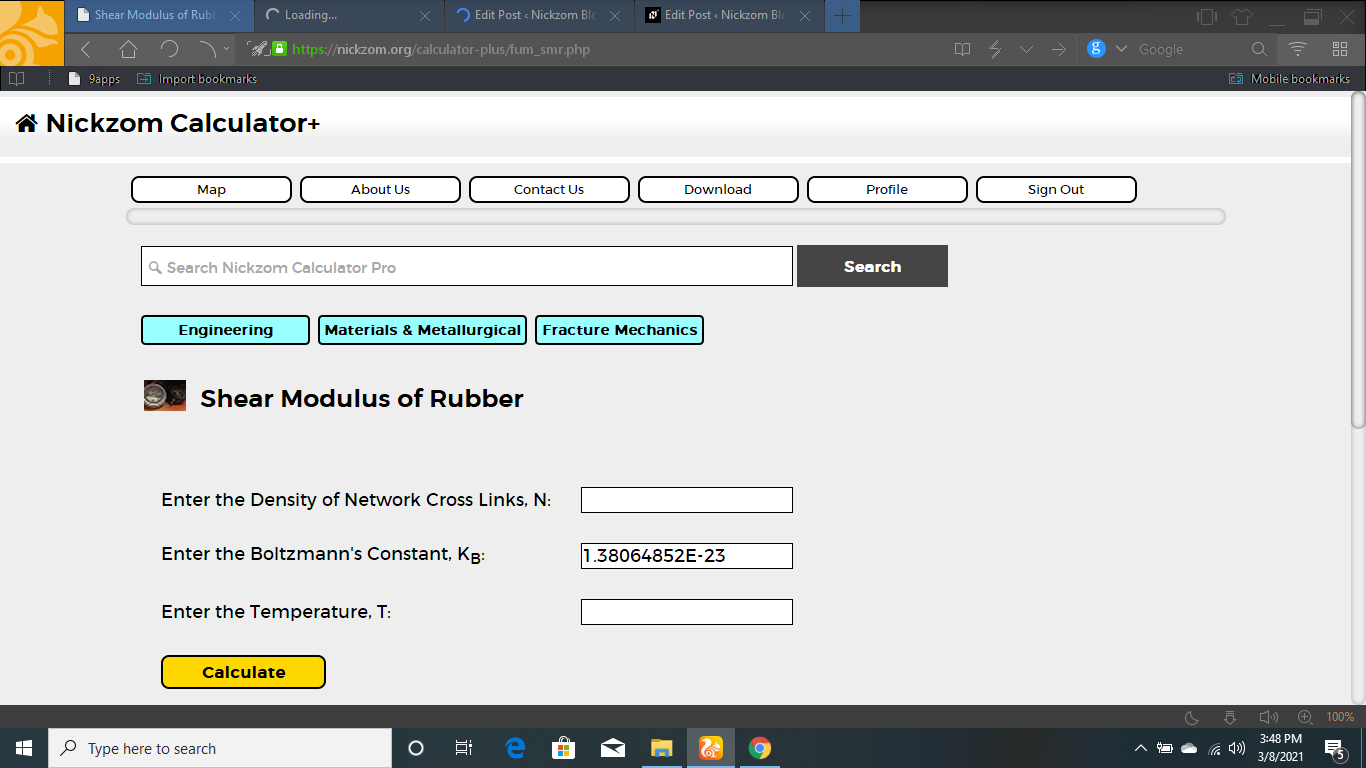Now, enter the value appropriately and accordingly for the parameter as required by the Density of Network Cross Links (N) is 21, Boltzmann’s Constant (KB) is 1.380e-23 and Temperature (T) is 10.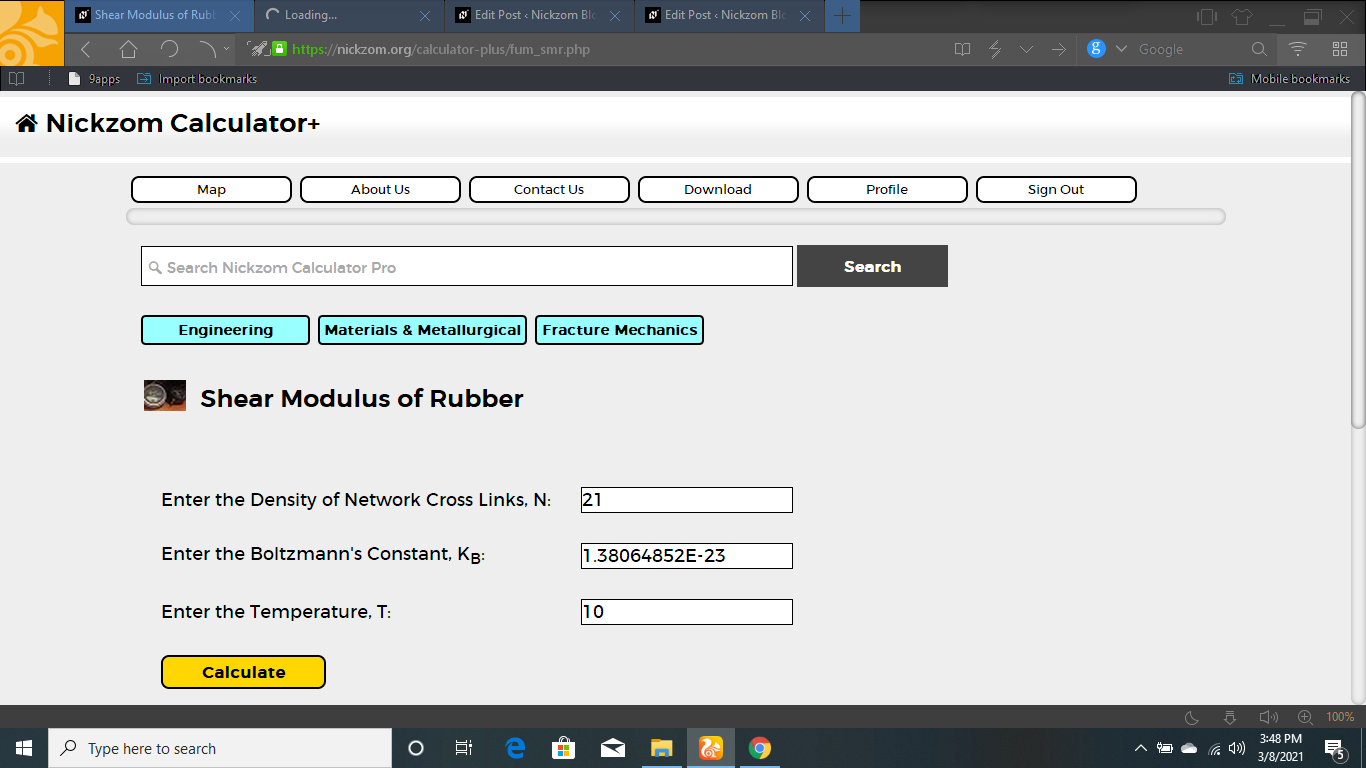Finally, Click on Calculate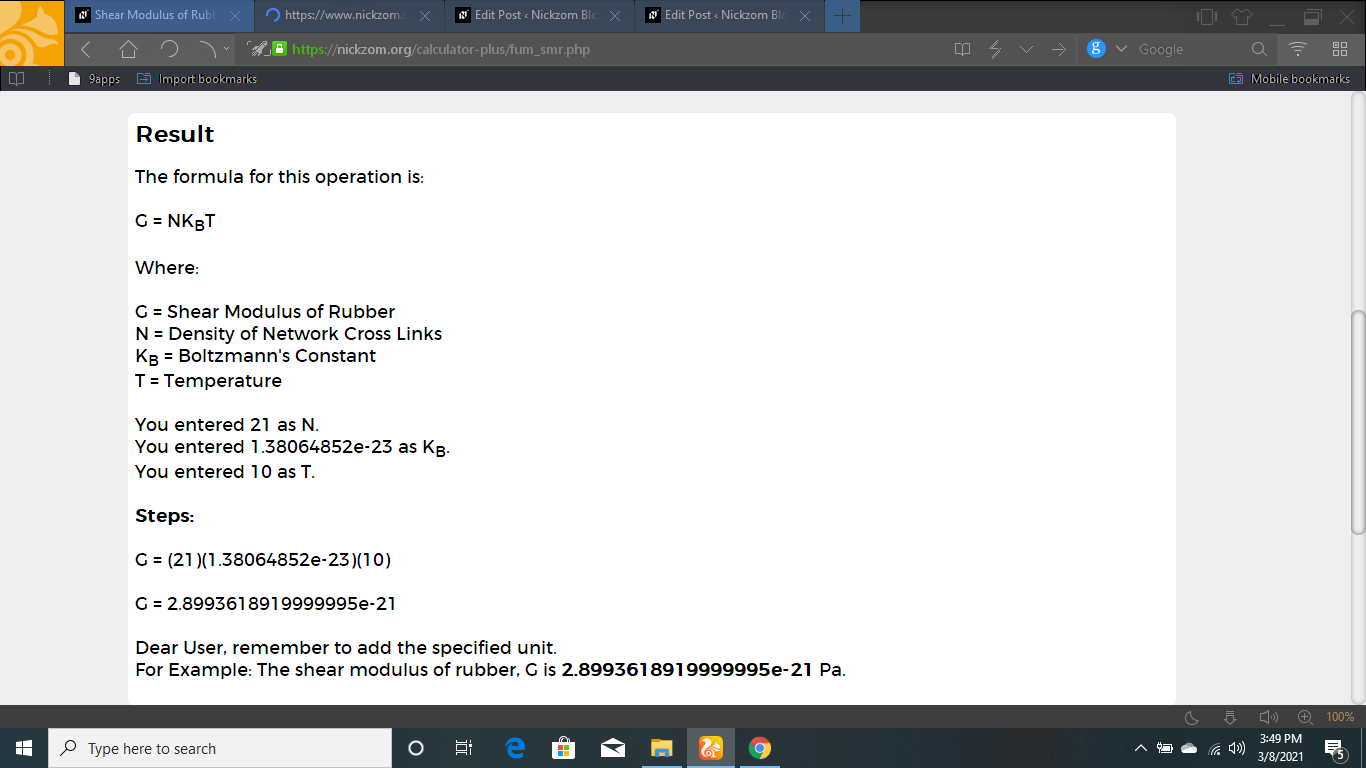As you can see from the screenshot above, Nickzom Calculator– The Calculator Encyclopedia solves for the shear modulus of rubber and presents the formula, workings and steps too.